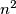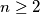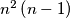### IMO Shortlist 1999 problem A2

Kvaliteta:
Avg: 0,0
Težina:
Avg: 6,0
The numbers from 1 to$n^2$ are randomly arranged in the cells of a$n \times n$ square ($n \geq 2$). For any pair of numbers situated on the same row or on the same column the ratio of the greater number to the smaller number is calculated. Let us call the characteristic of the arrangement the smallest of these$n^2\left(n-1\right)$ fractions. What is the highest possible value of the characteristic ?
Izvor: Međunarodna matematička olimpijada, shortlist 1999